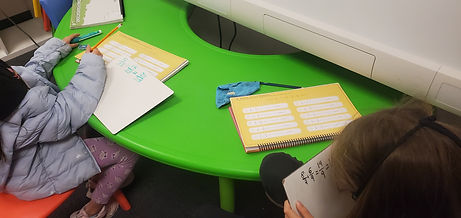Mr. Jake

Target 1​

Lesson Type:

Continuation

Number Operation

:

Fractional Numbers

Identity, compare, and manipulate different types of fractions.

1:

Convert improper fractions to mixed numbers (and vice versa), understanding that both have “wholes” as a part of their quantity.

2:

Understand that fractions with different numerators and denominators can represent the same size.

3:

Simplify a fraction to its lowest terms.

5th

Vocabulary:

Fraction, Numerator, Denominator.

Activities:

Determined the least common denominator of fractionsHome Exploration

Guiding Questions:Absent Students:

Target 2

:

1:

Accurately measure angles.

2:

Identify angles as acute, obtuse, or right.

3:

Identify angle types and estimate their measurement.

4:

Understand that angles are measured in degrees (from 0 to 360).

5:

Understand that an angle is the union of two rays with a common endpoint (vertex).

5th

Vocabulary:

Angle, Degree, Protractor, Right, Obtuse

Activities:

Practiced drawing and measuring angles using a protractor.

Determined if angles were right, acute, or obtuseHome Exploration

Guiding Questions:Target 3

:

Vocabulary:

Activities:Home Exploration